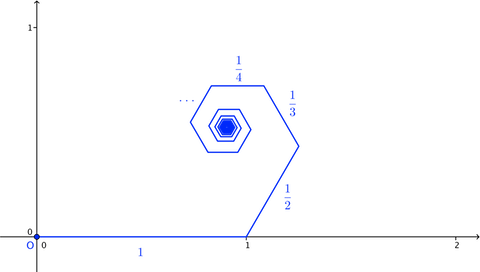# A Harmonic Hex

Calculus Level 2The image above shows a broken line (a series of connected line segments) starting at the origin, O. The nth segment in the broken line has length $\frac{1}{n}$, and at the end of each segment, the broken line turns $60^{\circ}$ counter-clockwise.

As the number of segments in the broken line approaches infinity, the final endpoint of the broken line approaches a point P. The distance OP can be written as $\frac{a}{b}\pi$, where a and b are positive coprime integers. Find $a+b$.

×